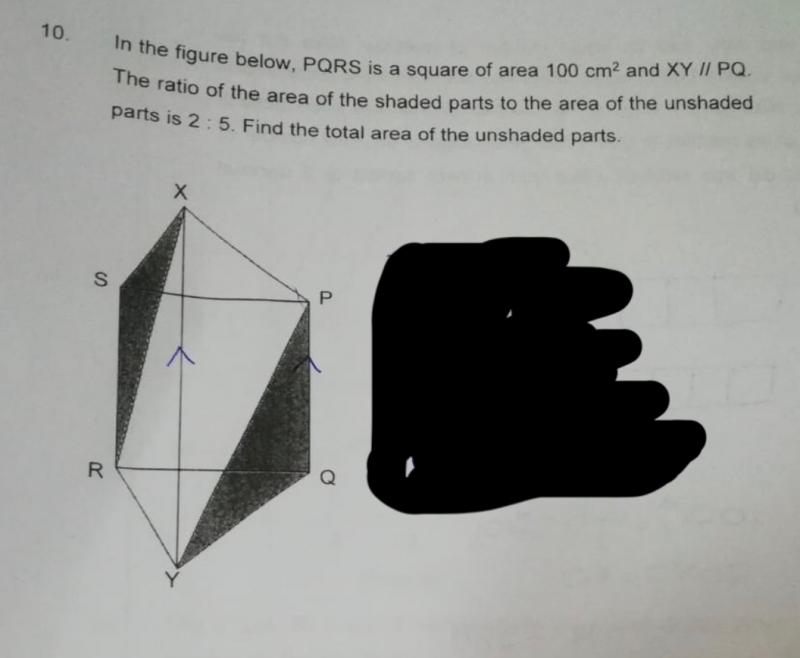# QuestionAppreciate any help on this question.

This is a Area question.

Ares of square = 100

Area of shaded = 100 ÷ 2 =50

2u = 50

1u = 25

5u = 5 × 25 = 125

Note

Move the point X to the line SP, you will get a right angle triangle.

Move the point Y to the line QR, you will get a right angle triangle.

These two triangles = half the square.